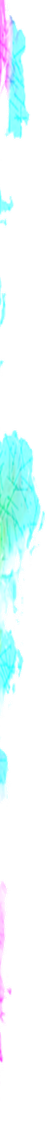### RegExp Conditional

• turned_in_notConditional
• x(?=y)
• x(?!y)
• To run below examples, try it inside `<script>` tag, in a basic html file

##### x(?=y)

It matches x only if x is followed by y

For example :

``````var str="10.101";
var pattern=/10(?=1)/g;
// Output: 10``````

Matches: 10.101

Comment : Match 10 which is followed by 1

##### x(?!y)

It matches x only if x is not followed by y

For example :

``````var str="10.101";
var pattern=/10(?!1)/g;